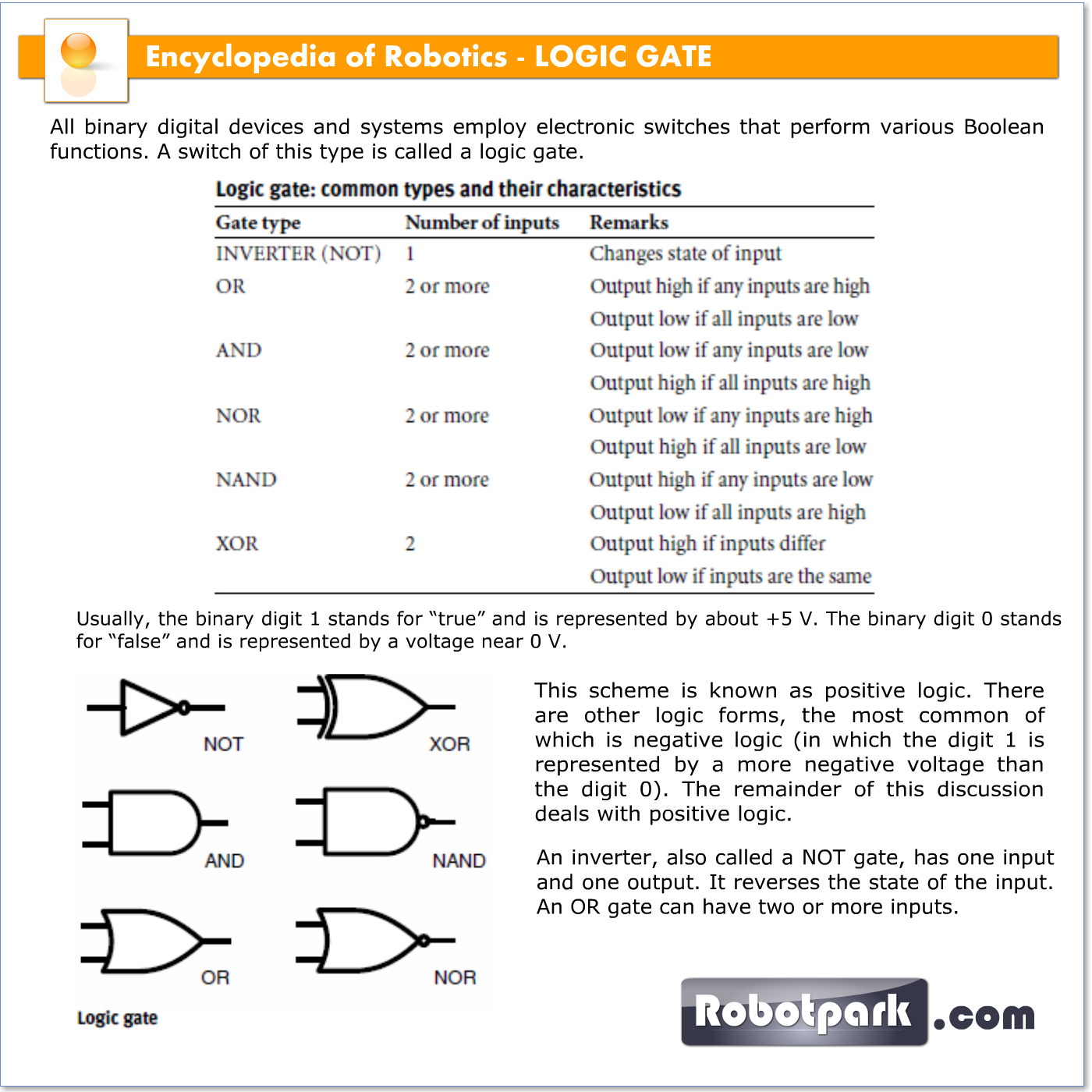# LOGIC GATE – 21083

All binary digital devices and systems employ electronic switches that perform various Boolean functions. A switch of this type is called a logic gate.

Usually, the binary digit 1 stands for “true” and is represented by about +5 V. The binary digit 0 stands for “false” and is represented by a voltage near 0 V. This scheme is known as positive logic. There are other logic forms, the most common of which is negative logic (in which the digit 1 is represented by a more negative voltage than the digit 0). The remainder of this discussion deals with positive logic.An inverter, also called a NOT gate, has one input and one output. It reverses the state of the input. An OR gate can have two or more inputs. If both, or all, of the inputs are 0, then the output is 0. If any of the inputs are 1, then the output is 1.An AND gate can have two or more inputs. If both, or all, of the inputs are 1, then the output is 1. Otherwise the output is 0.

Sometimes an inverter and an OR gate are combined. This produces a NOR gate. If an inverter and an AND gate are combined, the result is a NAND gate. An exclusive OR gate, also called an XOR gate, has two inputs and one output. If the two inputs are the same (either both 1 or both 0), then the output is 0. If the two inputs are different, then the output is 1. The functions of logic gates are summarized in the accompanying table, and their schematic symbols are shown in the illustration.